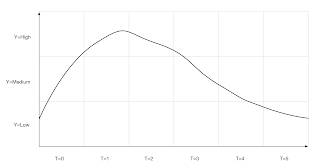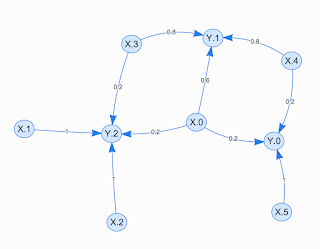## Wednesday, 27 January 2016

### How to convert "Time Series" to "Bayesian Network"

I already talked about time series in "Time series as a point in space" post and also discussed a way we can find its anomalies in "A simple way of calculating anomaly" post. But since I've been talking in recent posts about building Bayesian Network to learn existing patterns in information, here I want to show you how easily we can model a time series data with a Bayesian Network.

The process of converting a time series data to a network is nothing rather than the way we get information from a series when we look at it and build its pattern in our mind.

Look at the following time series, when you look at it, you don't need to know what this series is representing, or what are the unit of the Y or T axis. All gets your attention is the up and downs of the series through the time.Sample time series

So what you basically do in your mind is the following steps:

1- Building your imaginary Y and T axes with large units, like what you see in the picture.
2- Building a network in your mind which shows at your imaginary times, the possible features of the series.

For example, here in the picture, it simply shows that:

- At T=0, we could have Y value of "low", "medium" and "high", but the probability of having them is not equal. In fact, it is more likely to have it "medium" than the other 2 options.
- At T=1 and T=2, we have only "high" value for Y.
- At T=3, we have both "high" and "medium" but the probability of having "high" value is larger.
.
.
.

If you do this and draw the network, you'll have something like the following network, just note that the probability numbers are just an approximation and consider here instead of T-Y graph we have X-Y graph and instead of "low", "medium" and "high" we have 0, 1 and 2.Time series Bayesian Network

As you see in this graph we have our observation as a network of connected features with values. If you check it, you'll find it exactly complies what we observed before as for example at T=1 we only have Y="high" so here you see from node X.1 we have only the chance of going to node Y.2, or at X.0 we have chances of going to Y.0, Y1 and Y2 with different probabilities which somehow shows the curve and change pattern of the series during T=0.